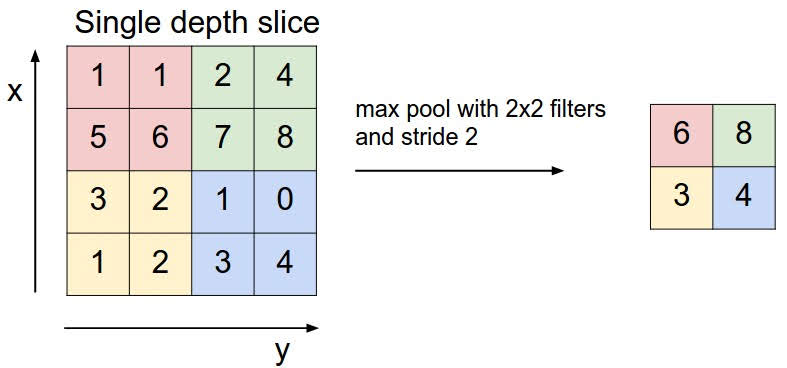# Pooling Layer

Categories:

Updated:

Pooling is extracting the most representative feature from each subset of input. By doing this, the height and width of the next layer unit decrease, so we can reduce computational cost and overfitting.

‘Max pooling’, which is the type of pooling we use the most, pulls out the maximum value within a particular subset. ‘Average pooling’, on the other hand, averages the values within a particular subset.In the above exmample, we are max pooling with 2x2 filters and stride 2. With pooling, we have reduced the unit size from 4x4 to 2x2.

In convolution layers, filter was 3-dimensional, so the output for one convolution was 2-dimensional. On the other hand, in pooling layers, pooling filter is 2-dimensional and applied to each channels of the input. So the output is still 3-dimensional.

Typically, we don’t use padding in pooling layers.

Also, there are no learnable parameters in pooling layers. Filter size, stride, and padding are hyperparameters that we manually set.

Categories: